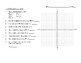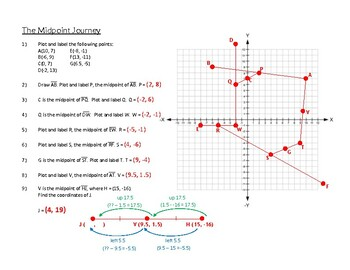# The Midpoint JourneySubject
Resource Type
File Type

PDF

(564 KB)
Product Rating
Standards
• Product Description
• StandardsNEW

Can the midpoint be found by simply counting the spaces? Will using rise and run help? Is the midpoint formula needed? In this activity, students will navigate through a series of graphed segments (segments they themselves graph) finding midpoints and endpoints. In each step, they’ll need to consider the best tool/approach. Students encounter easy questions where the midpoint can be found by simply counting the units on a horizontal line, for example. In some steps, students are given one endpoint and a midpoint and they must find the other endpoint. Not every point is made up of integers. Sometimes, the graph isn’t large enough to plot what they need (this is intentional).

I like this activity because students have to sort out for themselves which tool to use and when. It requires problems solving skills, creativity, as well as attention to detail. I do walk around and let them know when they are getting off track, as one wrong answer leads to everything that follows being incorrect as well.

Use appropriate tools strategically. Mathematically proficient students consider the available tools when solving a mathematical problem. These tools might include pencil and paper, concrete models, a ruler, a protractor, a calculator, a spreadsheet, a computer algebra system, a statistical package, or dynamic geometry software. Proficient students are sufficiently familiar with tools appropriate for their grade or course to make sound decisions about when each of these tools might be helpful, recognizing both the insight to be gained and their limitations. For example, mathematically proficient high school students analyze graphs of functions and solutions generated using a graphing calculator. They detect possible errors by strategically using estimation and other mathematical knowledge. When making mathematical models, they know that technology can enable them to visualize the results of varying assumptions, explore consequences, and compare predictions with data. Mathematically proficient students at various grade levels are able to identify relevant external mathematical resources, such as digital content located on a website, and use them to pose or solve problems. They are able to use technological tools to explore and deepen their understanding of concepts.
Solve problems involving scale drawings of geometric figures, including computing actual lengths and areas from a scale drawing and reproducing a scale drawing at a different scale.
Total Pages
N/A
Included
Teaching Duration
30 minutes
Report this Resource to TpT
Reported resources will be reviewed by our team. Report this resource to let us know if this resource violates TpT’s content guidelines.